# sklearn.decomposition.PCA¶

class sklearn.decomposition.PCA(n_components=None, *, copy=True, whiten=False, svd_solver='auto', tol=0.0, iterated_power='auto', random_state=None)[source]

Principal component analysis (PCA).

Linear dimensionality reduction using Singular Value Decomposition of the data to project it to a lower dimensional space. The input data is centered but not scaled for each feature before applying the SVD.

It uses the LAPACK implementation of the full SVD or a randomized truncated SVD by the method of Halko et al. 2009, depending on the shape of the input data and the number of components to extract.

It can also use the scipy.sparse.linalg ARPACK implementation of the truncated SVD.

Notice that this class does not support sparse input. See TruncatedSVD for an alternative with sparse data.

Read more in the User Guide.

Parameters
n_componentsint, float or ‘mle’, default=None

Number of components to keep. if n_components is not set all components are kept:

n_components == min(n_samples, n_features)


If n_components == 'mle' and svd_solver == 'full', Minka’s MLE is used to guess the dimension. Use of n_components == 'mle' will interpret svd_solver == 'auto' as svd_solver == 'full'.

If 0 < n_components < 1 and svd_solver == 'full', select the number of components such that the amount of variance that needs to be explained is greater than the percentage specified by n_components.

If svd_solver == 'arpack', the number of components must be strictly less than the minimum of n_features and n_samples.

Hence, the None case results in:

n_components == min(n_samples, n_features) - 1

copybool, default=True

If False, data passed to fit are overwritten and running fit(X).transform(X) will not yield the expected results, use fit_transform(X) instead.

whitenbool, default=False

When True (False by default) the components_ vectors are multiplied by the square root of n_samples and then divided by the singular values to ensure uncorrelated outputs with unit component-wise variances.

Whitening will remove some information from the transformed signal (the relative variance scales of the components) but can sometime improve the predictive accuracy of the downstream estimators by making their data respect some hard-wired assumptions.

svd_solver{‘auto’, ‘full’, ‘arpack’, ‘randomized’}, default=’auto’
If auto :

The solver is selected by a default policy based on X.shape and n_components: if the input data is larger than 500x500 and the number of components to extract is lower than 80% of the smallest dimension of the data, then the more efficient ‘randomized’ method is enabled. Otherwise the exact full SVD is computed and optionally truncated afterwards.

If full :

run exact full SVD calling the standard LAPACK solver via scipy.linalg.svd and select the components by postprocessing

If arpack :

run SVD truncated to n_components calling ARPACK solver via scipy.sparse.linalg.svds. It requires strictly 0 < n_components < min(X.shape)

If randomized :

run randomized SVD by the method of Halko et al.

New in version 0.18.0.

tolfloat, default=0.0

Tolerance for singular values computed by svd_solver == ‘arpack’. Must be of range [0.0, infinity).

New in version 0.18.0.

iterated_powerint or ‘auto’, default=’auto’

Number of iterations for the power method computed by svd_solver == ‘randomized’. Must be of range [0, infinity).

New in version 0.18.0.

random_stateint, RandomState instance or None, default=None

Used when the ‘arpack’ or ‘randomized’ solvers are used. Pass an int for reproducible results across multiple function calls. See Glossary.

New in version 0.18.0.

Attributes
components_ndarray of shape (n_components, n_features)

Principal axes in feature space, representing the directions of maximum variance in the data. The components are sorted by explained_variance_.

explained_variance_ndarray of shape (n_components,)

The amount of variance explained by each of the selected components. The variance estimation uses n_samples - 1 degrees of freedom.

Equal to n_components largest eigenvalues of the covariance matrix of X.

New in version 0.18.

explained_variance_ratio_ndarray of shape (n_components,)

Percentage of variance explained by each of the selected components.

If n_components is not set then all components are stored and the sum of the ratios is equal to 1.0.

singular_values_ndarray of shape (n_components,)

The singular values corresponding to each of the selected components. The singular values are equal to the 2-norms of the n_components variables in the lower-dimensional space.

New in version 0.19.

mean_ndarray of shape (n_features,)

Per-feature empirical mean, estimated from the training set.

Equal to X.mean(axis=0).

n_components_int

The estimated number of components. When n_components is set to ‘mle’ or a number between 0 and 1 (with svd_solver == ‘full’) this number is estimated from input data. Otherwise it equals the parameter n_components, or the lesser value of n_features and n_samples if n_components is None.

n_features_int

Number of features in the training data.

n_samples_int

Number of samples in the training data.

noise_variance_float

The estimated noise covariance following the Probabilistic PCA model from Tipping and Bishop 1999. See “Pattern Recognition and Machine Learning” by C. Bishop, 12.2.1 p. 574 or http://www.miketipping.com/papers/met-mppca.pdf. It is required to compute the estimated data covariance and score samples.

Equal to the average of (min(n_features, n_samples) - n_components) smallest eigenvalues of the covariance matrix of X.

n_features_in_int

Number of features seen during fit.

New in version 0.24.

See also

KernelPCA

Kernel Principal Component Analysis.

SparsePCA

Sparse Principal Component Analysis.

TruncatedSVD

Dimensionality reduction using truncated SVD.

IncrementalPCA

Incremental Principal Component Analysis.

References

For n_components == ‘mle’, this class uses the method from: Minka, T. P.. “Automatic choice of dimensionality for PCA”. In NIPS, pp. 598-604

Implements the probabilistic PCA model from: Tipping, M. E., and Bishop, C. M. (1999). “Probabilistic principal component analysis”. Journal of the Royal Statistical Society: Series B (Statistical Methodology), 61(3), 611-622. via the score and score_samples methods.

For svd_solver == ‘arpack’, refer to scipy.sparse.linalg.svds.

Examples

>>> import numpy as np
>>> from sklearn.decomposition import PCA
>>> X = np.array([[-1, -1], [-2, -1], [-3, -2], [1, 1], [2, 1], [3, 2]])
>>> pca = PCA(n_components=2)
>>> pca.fit(X)
PCA(n_components=2)
>>> print(pca.explained_variance_ratio_)
[0.9924... 0.0075...]
>>> print(pca.singular_values_)
[6.30061... 0.54980...]

>>> pca = PCA(n_components=2, svd_solver='full')
>>> pca.fit(X)
PCA(n_components=2, svd_solver='full')
>>> print(pca.explained_variance_ratio_)
[0.9924... 0.00755...]
>>> print(pca.singular_values_)
[6.30061... 0.54980...]

>>> pca = PCA(n_components=1, svd_solver='arpack')
>>> pca.fit(X)
PCA(n_components=1, svd_solver='arpack')
>>> print(pca.explained_variance_ratio_)
[0.99244...]
>>> print(pca.singular_values_)
[6.30061...]


Methods

 fit(X[, y]) Fit the model with X. fit_transform(X[, y]) Fit the model with X and apply the dimensionality reduction on X. Compute data covariance with the generative model. get_params([deep]) Get parameters for this estimator. Compute data precision matrix with the generative model. Transform data back to its original space. score(X[, y]) Return the average log-likelihood of all samples. Return the log-likelihood of each sample. set_params(**params) Set the parameters of this estimator. Apply dimensionality reduction to X.
fit(X, y=None)[source]

Fit the model with X.

Parameters
Xarray-like of shape (n_samples, n_features)

Training data, where n_samples is the number of samples and n_features is the number of features.

yIgnored
Returns
selfobject

Returns the instance itself.

fit_transform(X, y=None)[source]

Fit the model with X and apply the dimensionality reduction on X.

Parameters
Xarray-like of shape (n_samples, n_features)

Training data, where n_samples is the number of samples and n_features is the number of features.

yIgnored
Returns
X_newndarray of shape (n_samples, n_components)

Transformed values.

Notes

This method returns a Fortran-ordered array. To convert it to a C-ordered array, use ‘np.ascontiguousarray’.

get_covariance()[source]

Compute data covariance with the generative model.

cov = components_.T * S**2 * components_ + sigma2 * eye(n_features) where S**2 contains the explained variances, and sigma2 contains the noise variances.

Returns
covarray, shape=(n_features, n_features)

Estimated covariance of data.

get_params(deep=True)[source]

Get parameters for this estimator.

Parameters
deepbool, default=True

If True, will return the parameters for this estimator and contained subobjects that are estimators.

Returns
paramsdict

Parameter names mapped to their values.

get_precision()[source]

Compute data precision matrix with the generative model.

Equals the inverse of the covariance but computed with the matrix inversion lemma for efficiency.

Returns
precisionarray, shape=(n_features, n_features)

Estimated precision of data.

inverse_transform(X)[source]

Transform data back to its original space.

In other words, return an input X_original whose transform would be X.

Parameters
Xarray-like, shape (n_samples, n_components)

New data, where n_samples is the number of samples and n_components is the number of components.

Returns
X_original array-like, shape (n_samples, n_features)

Notes

If whitening is enabled, inverse_transform will compute the exact inverse operation, which includes reversing whitening.

score(X, y=None)[source]

Return the average log-likelihood of all samples.

See. “Pattern Recognition and Machine Learning” by C. Bishop, 12.2.1 p. 574 or http://www.miketipping.com/papers/met-mppca.pdf

Parameters
Xarray-like of shape (n_samples, n_features)

The data.

yIgnored
Returns
llfloat

Average log-likelihood of the samples under the current model.

score_samples(X)[source]

Return the log-likelihood of each sample.

See. “Pattern Recognition and Machine Learning” by C. Bishop, 12.2.1 p. 574 or http://www.miketipping.com/papers/met-mppca.pdf

Parameters
Xarray-like of shape (n_samples, n_features)

The data.

Returns
llndarray of shape (n_samples,)

Log-likelihood of each sample under the current model.

set_params(**params)[source]

Set the parameters of this estimator.

The method works on simple estimators as well as on nested objects (such as Pipeline). The latter have parameters of the form <component>__<parameter> so that it’s possible to update each component of a nested object.

Parameters
**paramsdict

Estimator parameters.

Returns
selfestimator instance

Estimator instance.

transform(X)[source]

Apply dimensionality reduction to X.

X is projected on the first principal components previously extracted from a training set.

Parameters
Xarray-like, shape (n_samples, n_features)

New data, where n_samples is the number of samples and n_features is the number of features.

Returns
X_newarray-like, shape (n_samples, n_components)

## Examples using sklearn.decomposition.PCA¶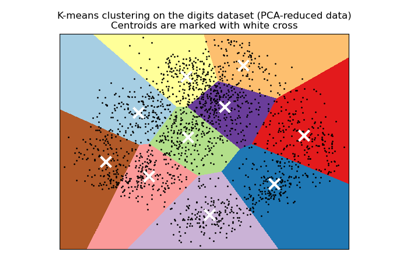A demo of K-Means clustering on the handwritten digits data¶Principal Component Regression vs Partial Least Squares Regression¶The Iris Dataset¶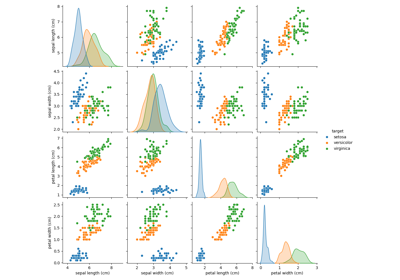PCA example with Iris Data-set¶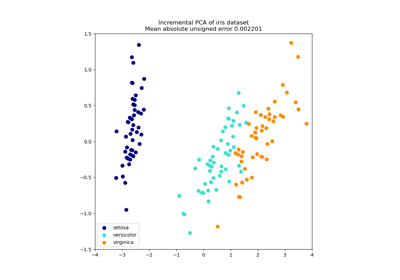Incremental PCA¶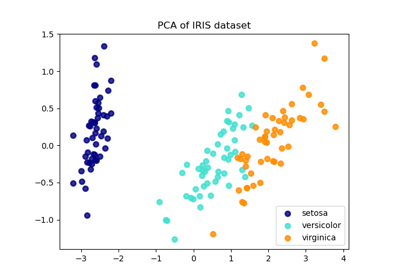Comparison of LDA and PCA 2D projection of Iris dataset¶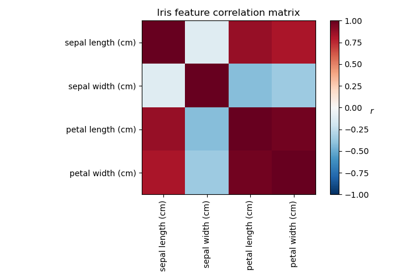Factor Analysis (with rotation) to visualize patterns¶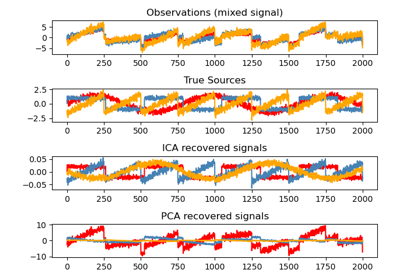Blind source separation using FastICA¶Principal components analysis (PCA)¶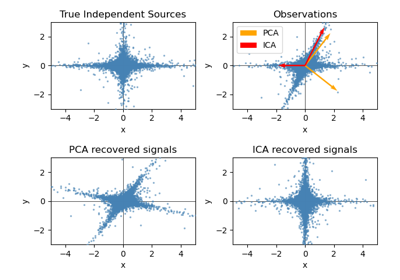FastICA on 2D point clouds¶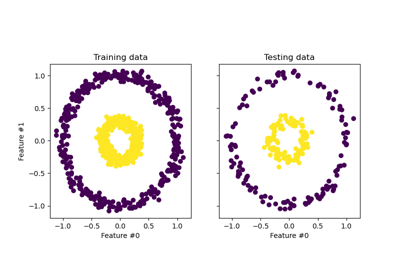Kernel PCA¶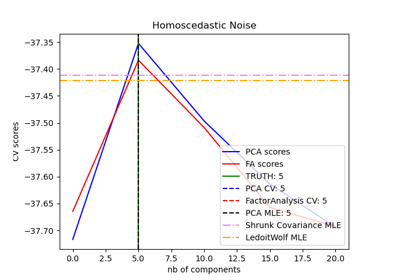Model selection with Probabilistic PCA and Factor Analysis (FA)¶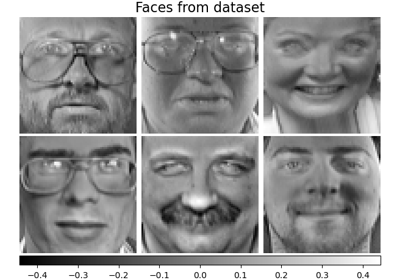Faces dataset decompositions¶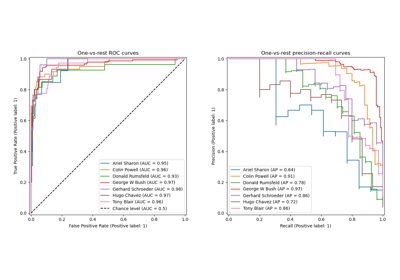Faces recognition example using eigenfaces and SVMs¶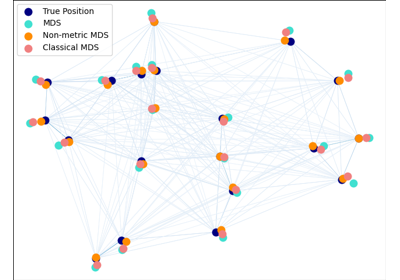Multi-dimensional scaling¶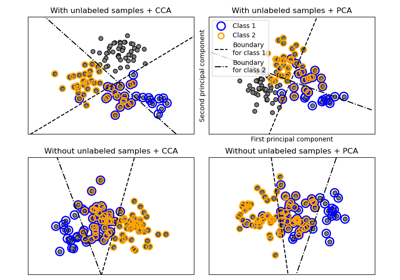Multilabel classification¶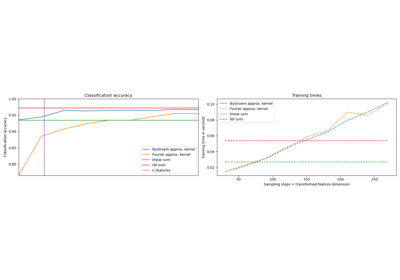Explicit feature map approximation for RBF kernels¶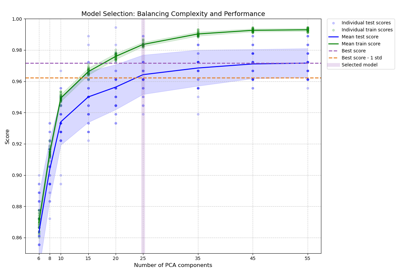Balance model complexity and cross-validated score¶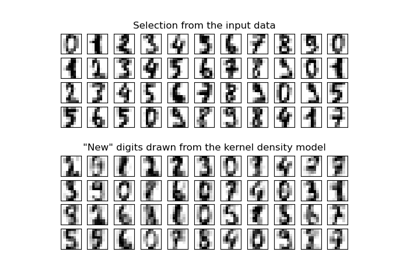Kernel Density Estimation¶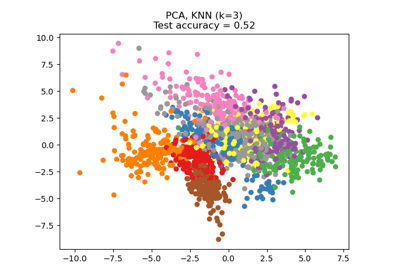Dimensionality Reduction with Neighborhood Components Analysis¶Concatenating multiple feature extraction methods¶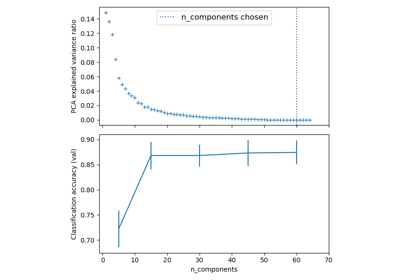Pipelining: chaining a PCA and a logistic regression¶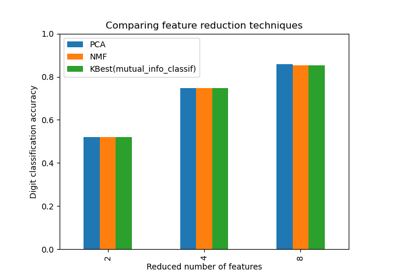Selecting dimensionality reduction with Pipeline and GridSearchCV¶Importance of Feature Scaling¶